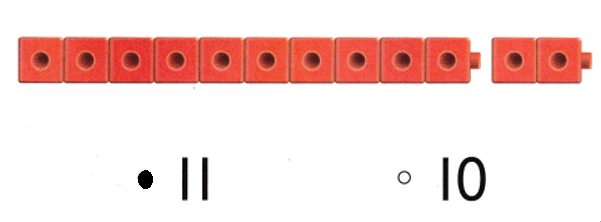# Texas Go Math Kindergarten Lesson 7.6 Answer Key One More and One Less

Refer to our Texas Go Math Kindergarten Answer Key Pdf to score good marks in the exams. Test yourself by practicing the problems from Texas Go Math Kindergarten Lesson 7.6 Answer Key One More and One Less.

## Texas Go Math Kindergarten Lesson 7.6 Answer Key One More and One Less

Unlock the Problem

DIRECTIONS: There are 14 children in the classroom. One more child walks into the classroom. How many children are in the classroom now? Draw to solve the problem. Write the number.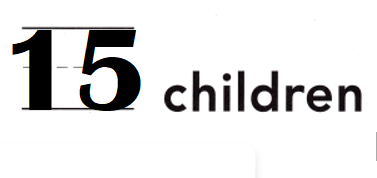Explanation:
Number of children in the classroom = 14.
Number of children added in the classroom = 1.
Total number of children are in the classroom now = Number of children in the classroom + Number of children added in the classroom
= 14 + 1
= 15.

Try Another Problem
DIRECTIONS: 1. Cole has 13 marbles. Phillip has one fewer marble than Cole. How many marbles does Phillip have? Draw to solve the problem. Write the number.

Question 1.Explanation:
Number of marbles Cole has = 13.
Phillip has one fewer marble than Cole.
=> Number of marbles Phillips has = Number of marbles Cole has – 1
= 13 – 1
= 12 or Twelve.

Share and Show
DIRECTIONS: 2. There are 12 children in the drink line. The snack line has one more child than the drink line. How many children are in the snack line? Draw to solve the problem. Write the number.

Question 2.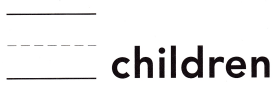Explanation:
Number of children in the drink line = 12.
The snack line has one more child than the drink line.
=> Number of children  in the snack line = Number of children in the drink line + 1
= 12 + 1
= 13 or Thirteen.

HOME ACTIVITY • Use drawing paper to hove your child draw pictures of one more or one less than any number 1 to 15.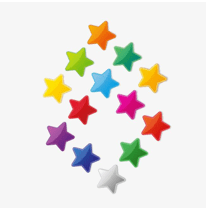Explanation:
Mt kid drawn different colors of stars.
Number of stars = 13 or Thirteen.

3. Choose the correct answer. The bakery has 13 muffins. Which set shows a number one less than 13? 4. Choose the correct answer. There are 13 red cubes. What number is one more?

Question 3.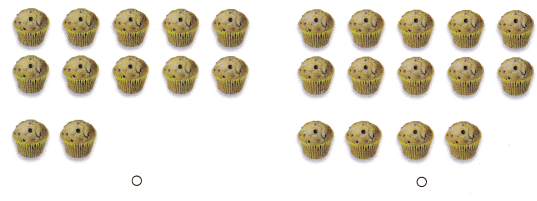Explanation:
Number of muffins less than 13 = 13 – 1 = 12 or Twelve.

Question 4.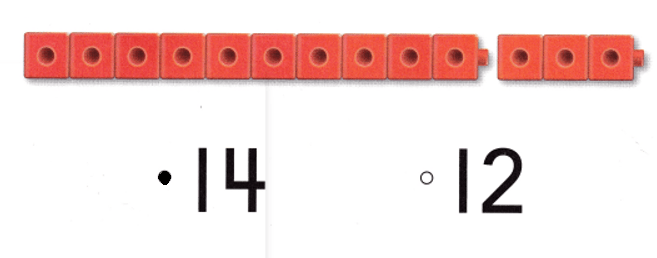Explanation:
Number of red cubes = 13.
Number of muffins more than 13 = 13 + 1 = 14 or Fourteen.

### Texas Go Math Kindergarten Lesson 7.6 Homework and Practice Answer Key

DIRECTIONS: 1. There are 15 dogs playing at the dog park. One dog goes home. How many dogs are playing at the dog park now? Draw to solve the problem. Write the number.

Question 1.Explanation:
Number of dogs playing at the dog park = 15.
Number of dogs goes home = 1.
Number of  dogs are playing at the dog park now = Number of dogs playing at the dog park – Number of dogs goes home
= 15 – 1
= 14 or Fourteen.

DIRECTIONS: Choose the correct answer. 2. Lisa has 14 bear counters. Which set shows a number one more than 14? 3. There are 12 red cubes. What number is one less?

Lesson Check
Question 2.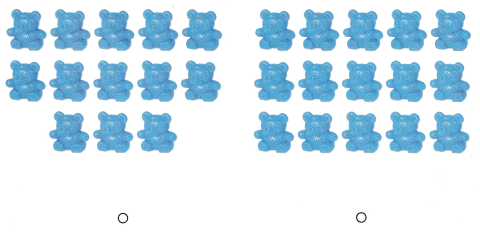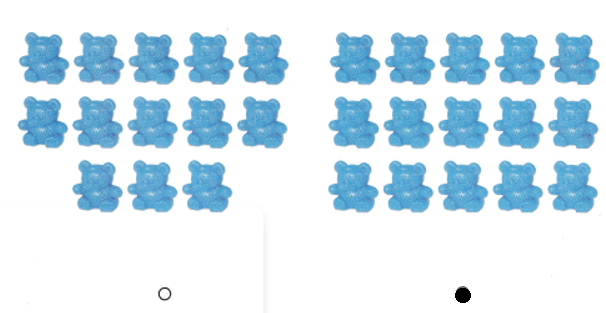Explanation:
Number of bear counters Lisa has = 14.
Set shows a number one more than 14 = 14 + 1 = 15 or Fifteen.

Question 3.